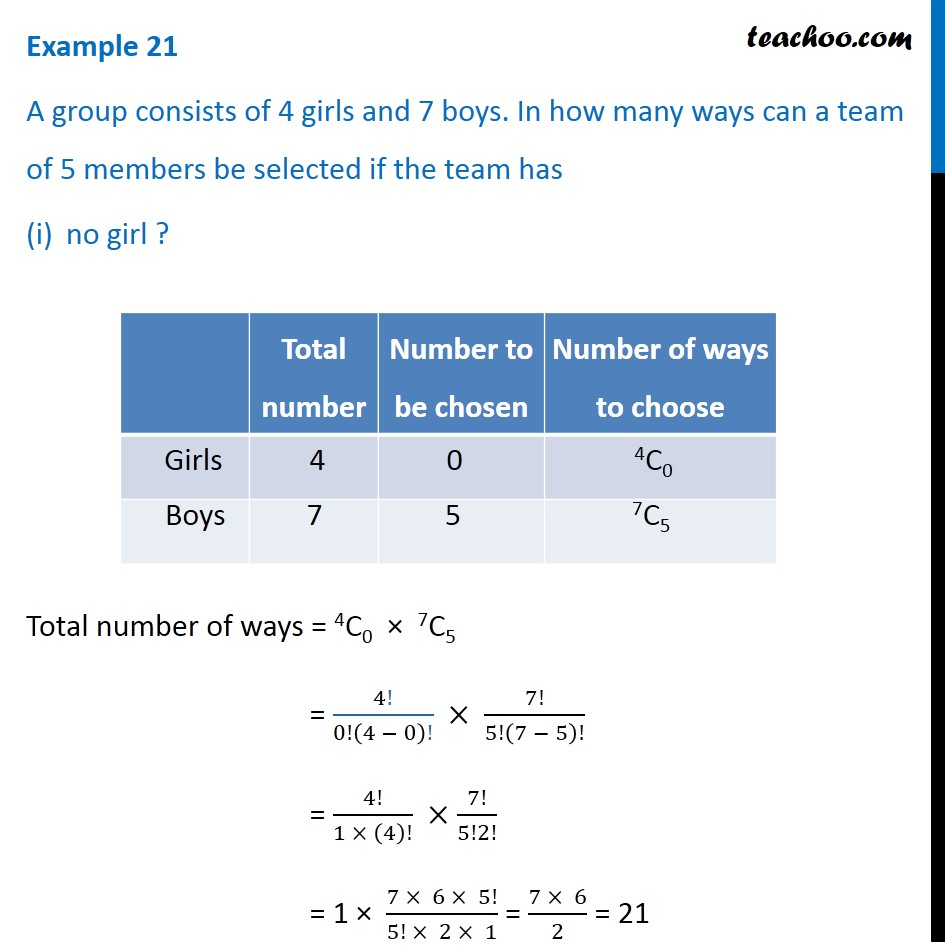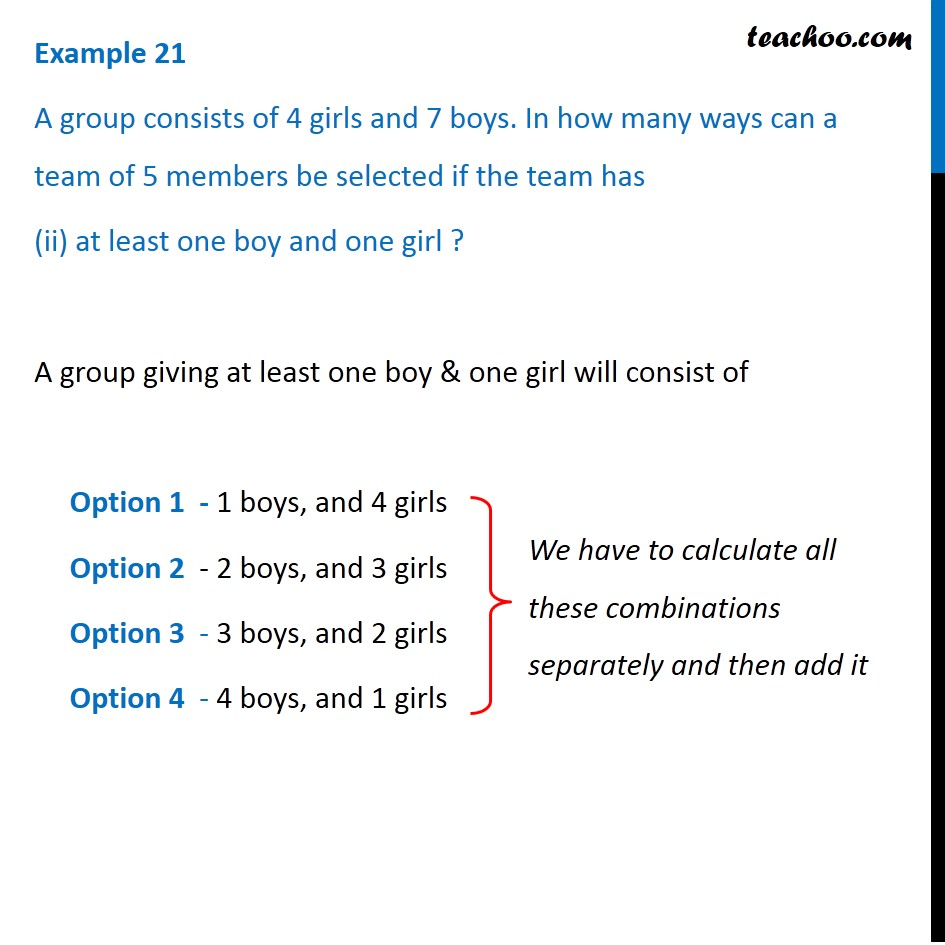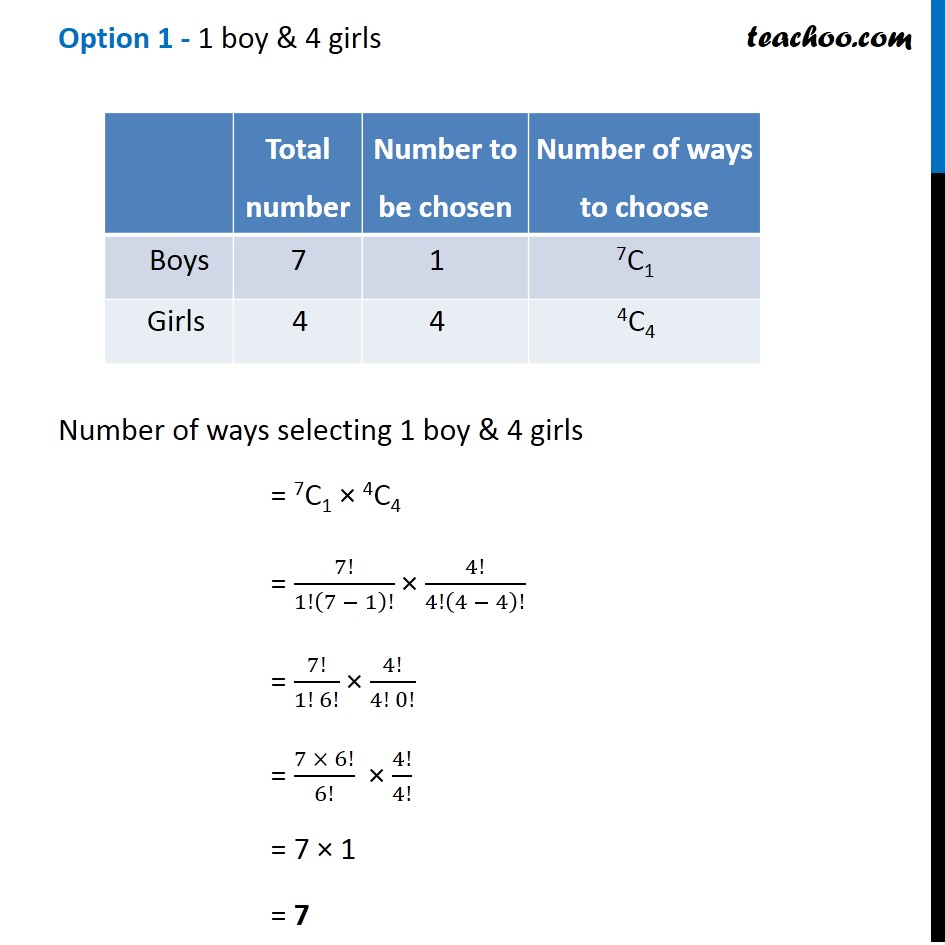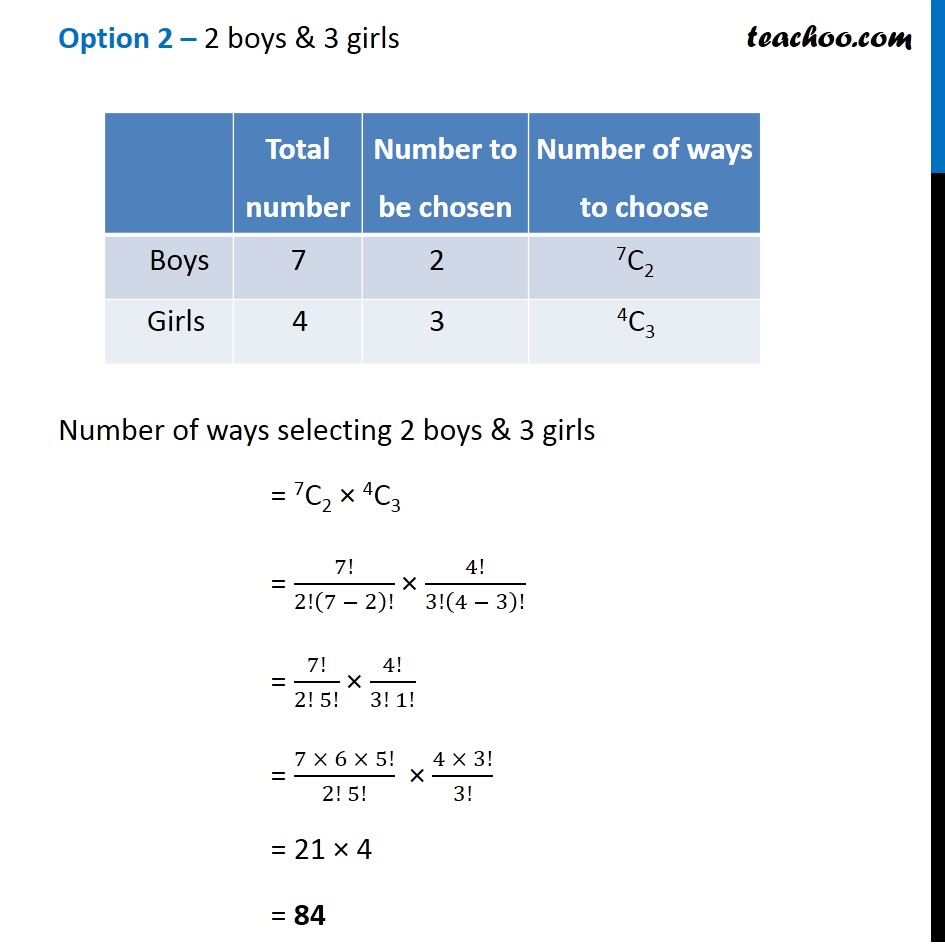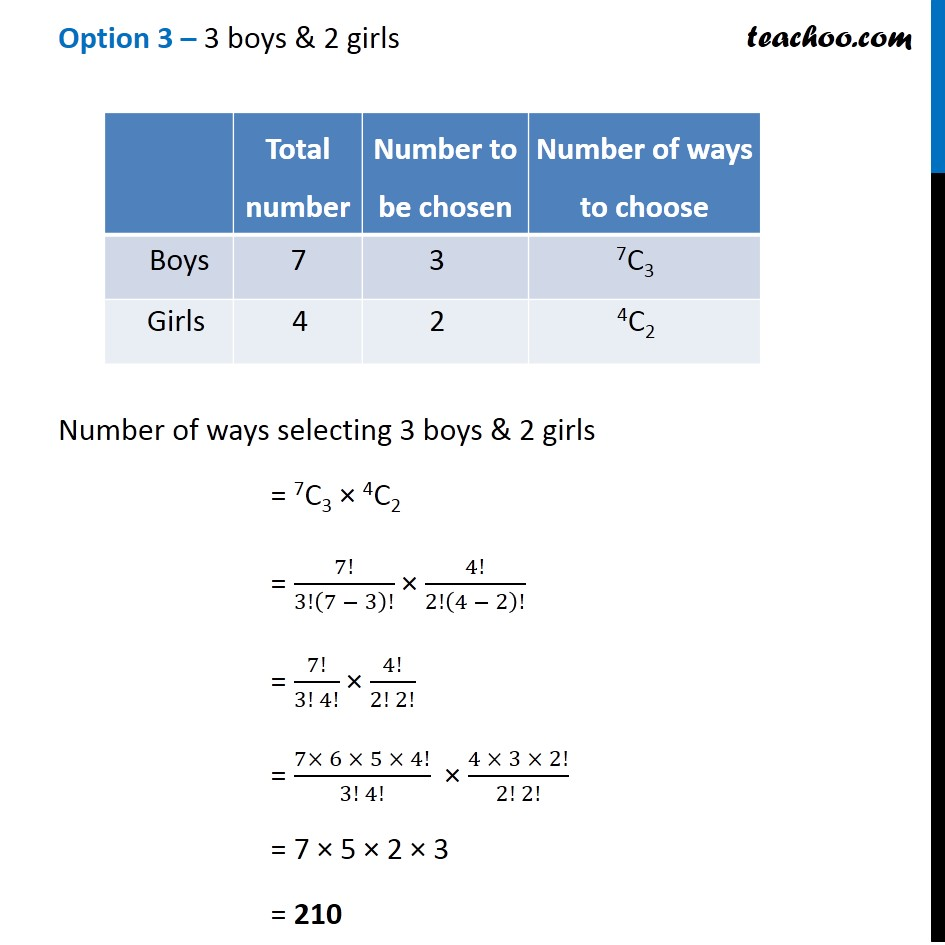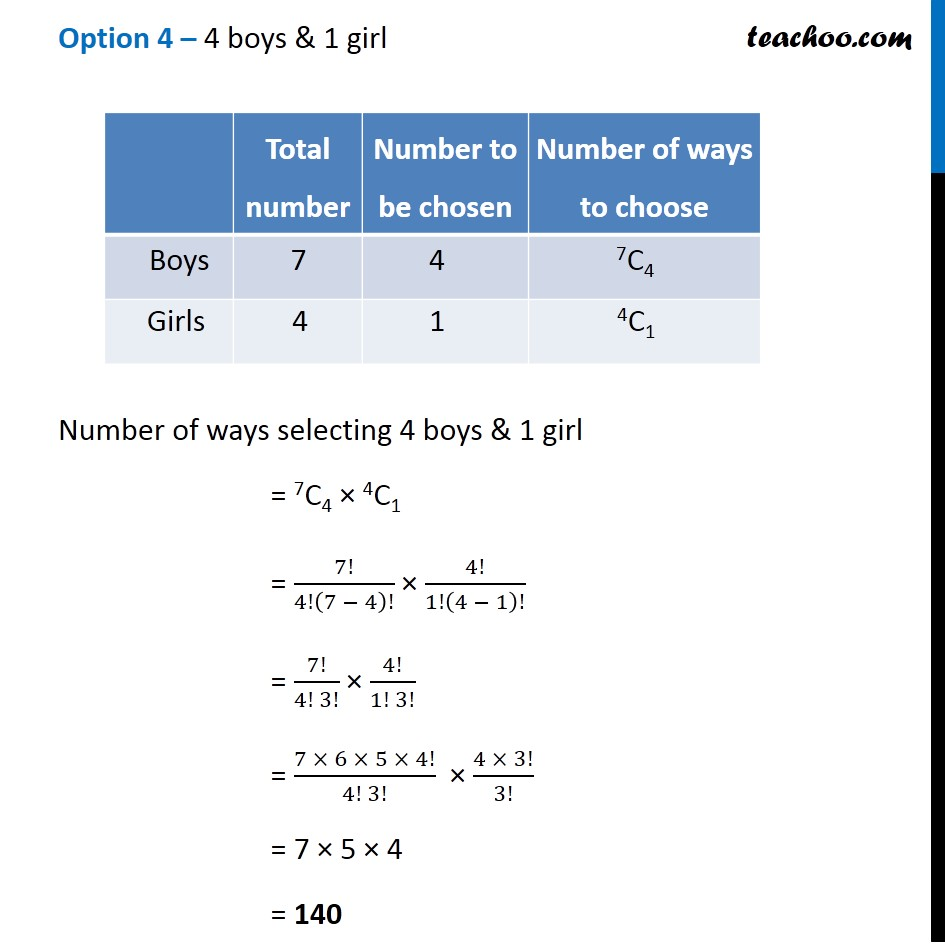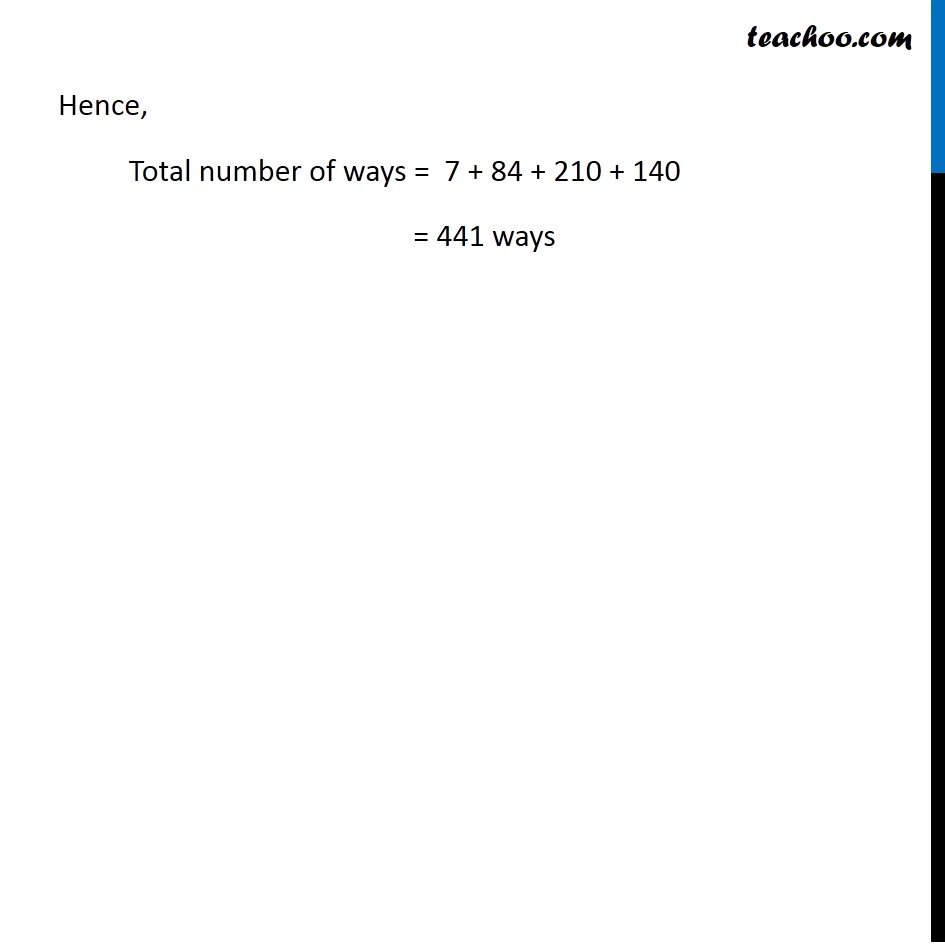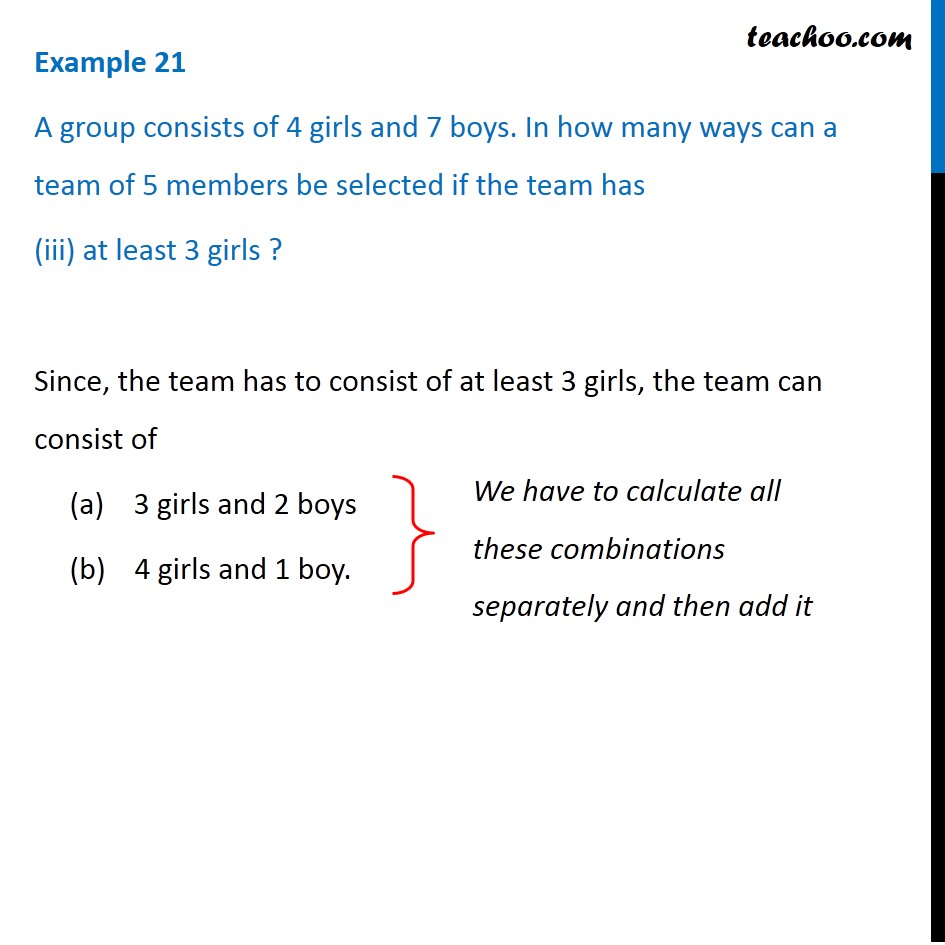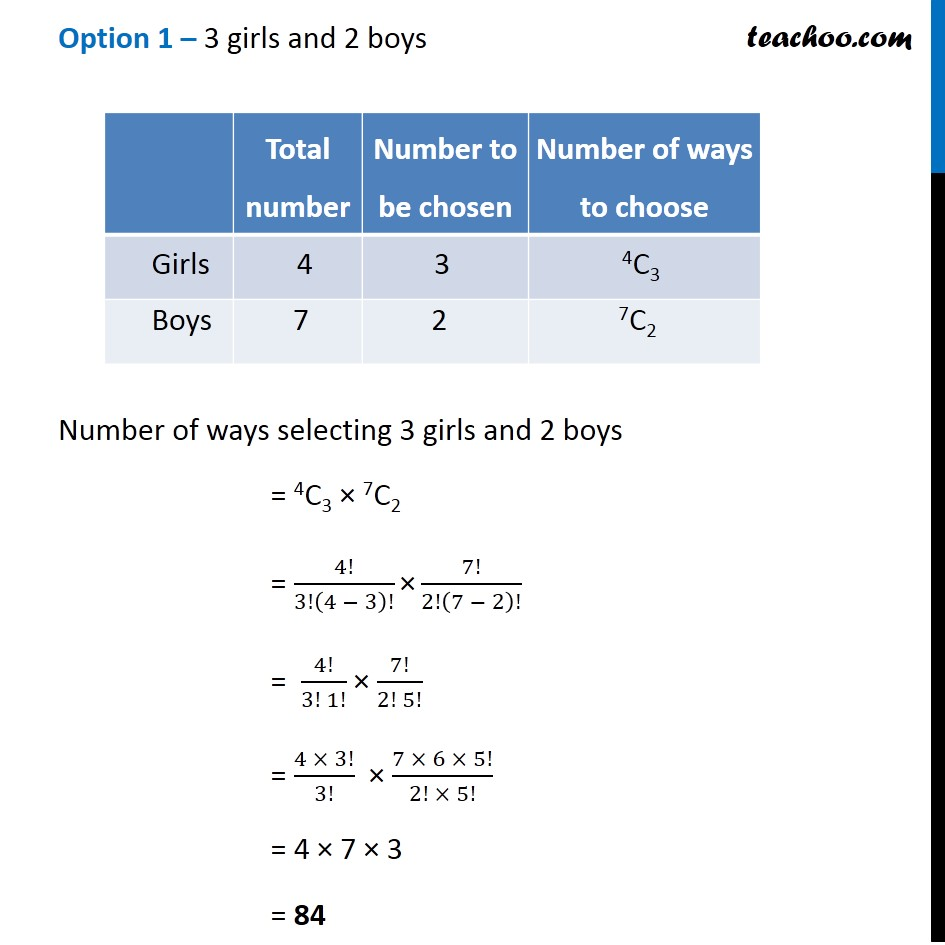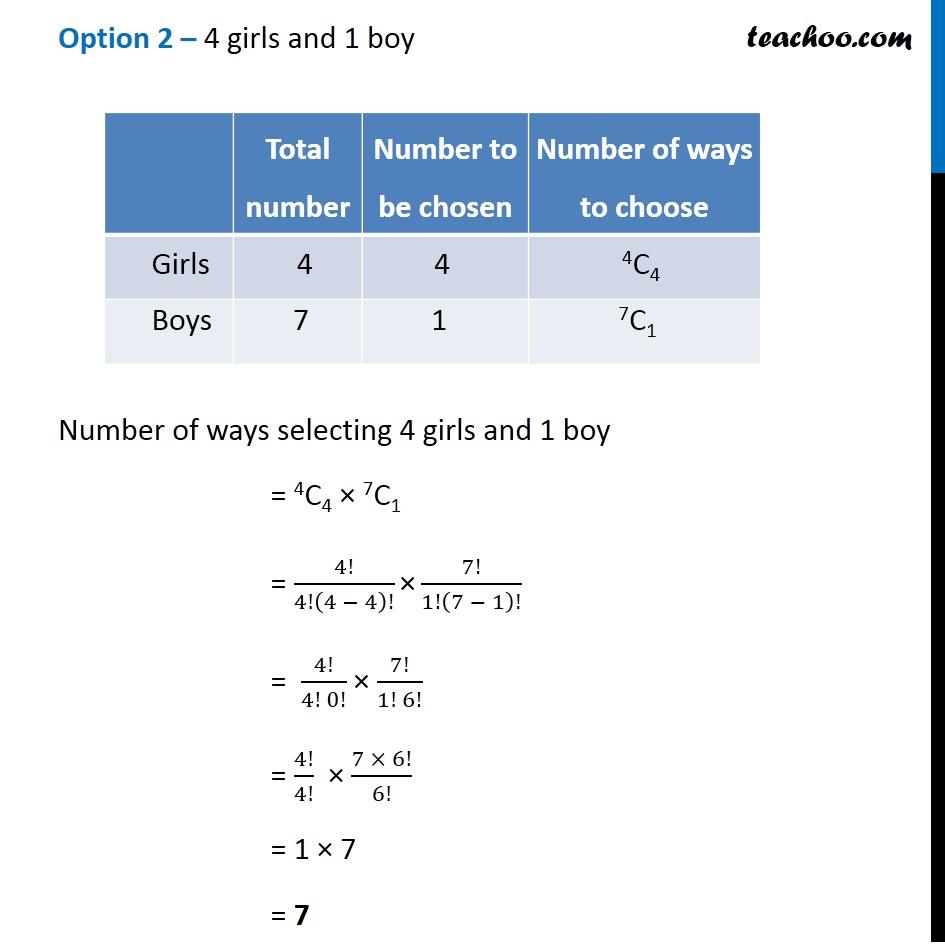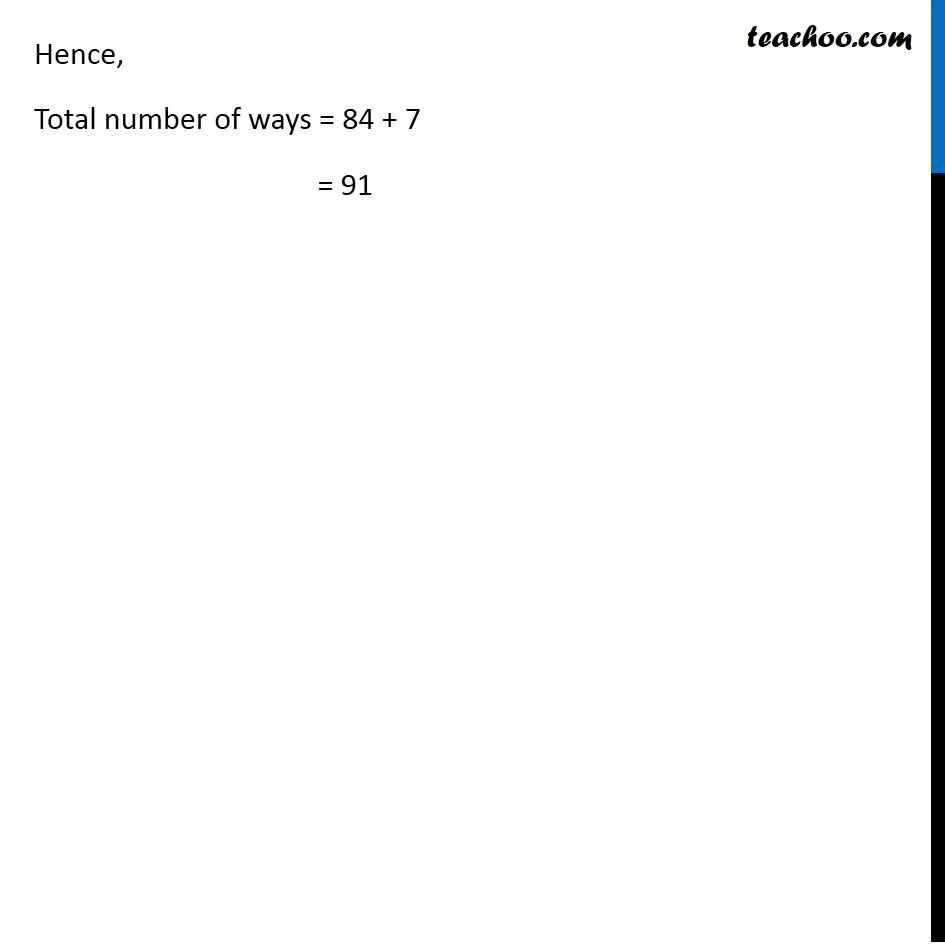Subscribe to our Youtube Channel - https://you.tube/teachoo

1. Chapter 7 Class 11 Permutations and Combinations
2. Serial order wise
3. Examples

Transcript

Example 21 A group consists of 4 girls and 7 boys. In how many ways can a team of 5 members be selected if the team has no girl ? Total number of ways = 4C0 × 7C5 = 4!/0!(4 − 0)! × 7!/5!(7 − 5)! = 4!/(1 × (4)!) ×7!/5!2! = 1 × (7 × 6 × 5!)/(5! × 2 × 1) = (7 × 6)/2 = 21 Example 21 A group consists of 4 girls and 7 boys. In how many ways can a team of 5 members be selected if the team has (ii) at least one boy and one girl ? A group giving at least one boy & one girl will consist of Option 1 - 1 boys, and 4 girls Option 2 - 2 boys, and 3 girls Option 3 - 3 boys, and 2 girls Option 4 - 4 boys, and 1 girls We have to calculate all these combinations separately and then add it Option 1 - 1 boy & 4 girls Number of ways selecting 1 boy & 4 girls = 7C1 × 4C4 = 7!/1!(7 − 1)! × 4!/4!(4 − 4)! = 7!/(1! 6!) × 4!/(4! 0!) = (7 × 6!)/6! × 4!/4! = 7 × 1 = 7 Option 2 – 2 boys & 3 girls Number of ways selecting 2 boys & 3 girls = 7C2 × 4C3 = 7!/2!(7 − 2)! × 4!/3!(4 − 3)! = 7!/(2! 5!) × 4!/(3! 1!) = (7 × 6 × 5!)/(2! 5!) × (4 × 3!)/3! = 21 × 4 = 84 Option 3 – 3 boys & 2 girls Number of ways selecting 3 boys & 2 girls = 7C3 × 4C2 = 7!/3!(7 − 3)! × 4!/2!(4 − 2)! = 7!/(3! 4!) × 4!/(2! 2!) = (7× 6 × 5 × 4!)/(3! 4!) × (4 × 3 × 2!)/(2! 2!) = 7 × 5 × 2 × 3 = 210 Option 4 – 4 boys & 1 girl Number of ways selecting 4 boys & 1 girl = 7C4 × 4C1 = 7!/4!(7 − 4)! × 4!/1!(4 − 1)! = 7!/(4! 3!) × 4!/(1! 3!) = (7 × 6 × 5 × 4!)/(4! 3!) × (4 × 3!)/3! = 7 × 5 × 4 = 140 Hence, Total number of ways = 7 + 84 + 210 + 140 = 441 ways Example 21 A group consists of 4 girls and 7 boys. In how many ways can a team of 5 members be selected if the team has (iii) at least 3 girls ? Since, the team has to consist of at least 3 girls, the team can consist of 3 girls and 2 boys 4 girls and 1 boy. We have to calculate all these combinations separately and then add it Option 1 – 3 girls and 2 boys Number of ways selecting 3 girls and 2 boys = 4C3 × 7C2 = 4!/3!(4 − 3)! "×" 7!/2!(7 − 2)! = 4!/(3! 1!) × 7!/(2! 5!) = (4 × 3!)/3! × (7 × 6 × 5!)/(2! × 5!) = 4 × 7 × 3 = 84 Option 2 – 4 girls and 1 boy Number of ways selecting 4 girls and 1 boy = 4C4 × 7C1 = 4!/4!(4 − 4)! "×" 7!/1!(7 − 1)! = 4!/(4! 0!) × 7!/(1! 6!) = 4!/4! × (7 × 6!)/6! = 1 × 7 = 7 Hence, Total number of ways = 84 + 7 = 91

Examples